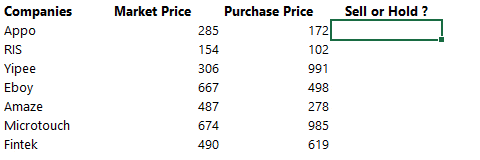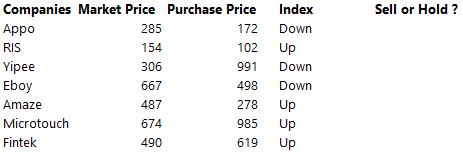Today, let me tell you a couple of interesting things about the IF function and its different variations

#### The IF Function

The IF function is one of the most elementary and important functions in the kitty of logical functions. Let’s try and explore this a bit

Here is a simple English statement : “If it rains today, then I will not go to work, else I will go to work”

Notice the 3 parts If, Then and Else. Just as this simple statement has 3 parts so does the IF formula

=IF ( CONDITION, ACTION IF TRUE, ACTION IF FALSE)

Parts of IF formula

• Condition – The first part is writing the condition you want to check for eg raining or not? The answer to that could be TRUE or FALSE
• Action if Condition is TRUE – In the second part what action will you take if the condition is TRUE, for eg “not go to work”
• Action if Condition is FALSE – In the third part what action will you take if the condition is FALSE, for eg “go to work”. This part is not mandatory, if you leave it blank excel returns FALSE

Let’s try and apply this to a more profound problem

Stock Buy Sell Example – The condition is,  Sell if you are making any profit, else HoldLet’s write an IF logic to do it ? It is simple just write the 3 parts of IF : Condition, Action if TRUE, Action if FALSEThe formula is  =IF(Market Price > Purchase Price, “Sell” ,”Hold”)

• Condition: If there is Profit (MP> PP)
• Action if True : Then Sell. Put “Sell” in double quotes because that is a text
• Action if False : Else Hold

#### The Nested IF

Let’s go a step further in the problemTake a look at the above case, we have also added market Index. Here is what I want you to do: If the stock is profitable, sell it else check the market index, If the market is going Down, sell it else hold

Note that I am asking you to check 2 things: profitability and then check market index (if the stock is not profitable). Here is how we can solve this with a Nested IF (if inside another if)The formula is pretty simple! Notice the 3 parts of IF and the Nested IFA General Rule for Nested IF

• Whenever you are dealing with subsequent logic : meaning one logic is applied subsequent to another logic, you are most likely headed towards nested if.
• In our example if we were making a loss then we checked the market index (i.e. market index up or down to be checked subsequent to profitability check)

#### The AND Function

Okay one more variation to the same problemIn the above example buy those stocks which are profitable and market is up. Notice that we need 2 conditions true at the same time i.e. profitability and market = up.

On the other hand the IF function allows you to write just one condition at a time, so we’ve got to use AND function (for checking 2 or more conditions simultaneously)Here is the explanation of the AND formulaNote a few things about the AND Function

1. The AND function can accept 255 conditions in it, separated by a commas
2. If all the conditions inside AND are true, it returns TRUE else FALSE
3. AND alone is not capable of taking action, if conditions are true so we often wrap it around the IF function to do something if the AND is returning a TRUE or FALSE
4. Whenever you want 2 or more conditions to be checked simultaneously use an AND function

#### Finally BOOLEAN Logic

If you google the term Boolean Logic you can get to know in depth about it, but in simple words a Boolean in Excel means

• TRUE = 1
• FALSE = 0

Sounds simple but it has an incredible utility and can drastically shorten the formulas. Let’s tweak the AND example for an instanceCalculate the profit of the stocks that are profitable and where market is up.

• Again 2 conditions at the same time so we will use AND
• But this time we have to calculate the profit of the stocks that we are buying, instead of writing the word “Buy”

Let’s try Boolean Logic on this• The AND function is the same as previous example, it returns either a TRUE or FALSE
• Remember TRUE =1 and FALSE = 0
• Then the AND formula is multiplied with Profit (which is MP – PP)
• If the AND returns FALSE or a 0. Zero x any number = 0
• If the AND return TRUE or a 1. One x any number = the same number

Boolean logic works beautifully when you have to use the TRUE or FALSE to further calculate something